# Solubility EquilibriaPage 1

#### WATCH ALL SLIDES

Slide 1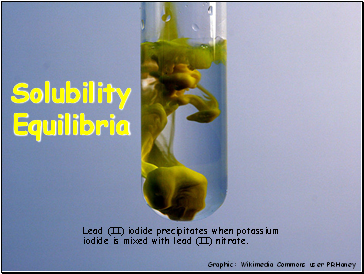## Solubility Equilibria

Lead (II) iodide precipitates when potassium iodide is mixed with lead (II) nitrate.

Graphic: Wikimedia Commons user PRHaney

Slide 2Ksp Values for Some Salts at 25C

Slide 3## Solving Solubility Problems

For the salt AgI at 25C, Ksp = 1.5 x 10-16

AgI(s)  Ag+(aq) + I-(aq)

O

O

+x

+x

x

x

1.5 x 10-16 = x2

x = solubility of AgI in mol/L = 1.2 x 10-8 M

Slide 4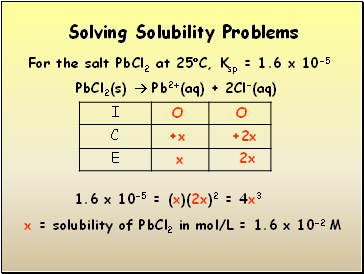Solving Solubility Problems

For the salt PbCl2 at 25C, Ksp = 1.6 x 10-5

PbCl2(s)  Pb2+(aq) + 2Cl-(aq)

O

O

+x

+2x

x

2x

1.6 x 10-5 = (x)(2x)2 = 4x3

x = solubility of PbCl2 in mol/L = 1.6 x 10-2 M

Slide 5## Solving Solubility with a Common Ion

For the salt AgI at 25C, Ksp = 1.5 x 10-16

What is its solubility in 0.05 M NaI?

AgI(s)  Ag+(aq) + I-(aq)

0.05

O

+x

0.05+x

x

0.05+x

1.5 x 10-16 = (x)(0.05+x)  (x)(0.05)

x = solubility of AgI in mol/L = 3.0 x 10-15 M

Slide 6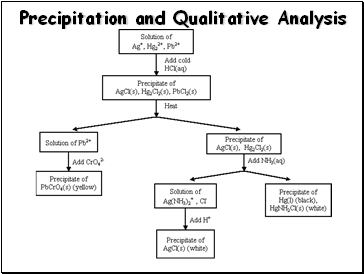## Precipitation and Qualitative Analysis

Slide 7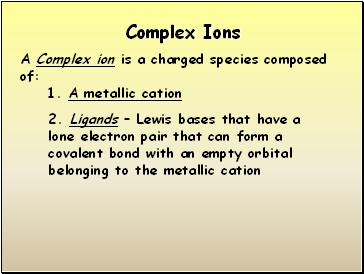## Complex Ions

A Complex ion is a charged species composed of:

1. A metallic cation

2. Ligands – Lewis bases that have a lone electron pair that can form a covalent bond with an empty orbital belonging to the metallic cation

Slide 8NH3, CN-, and H2O are Common Ligands

Slide 9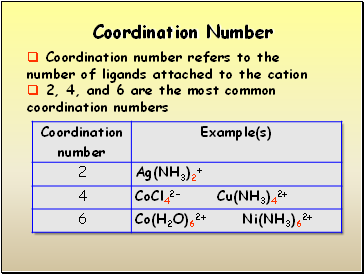## Coordination Number

Coordination number refers to the number of ligands attached to the cation

2, 4, and 6 are the most common coordination numbers

Slide 10## Complex Ions and Solubility

AgCl(s)  Ag+ + Cl- Ksp = 1.6 x 10-10

Ag+ + NH3  Ag(NH3)+ K1 = 2.1 x 103

Ag(NH3)+ NH3  Ag(NH3)2+ K2 = 8.2 x 103

AgCl + 2NH3  Ag(NH3)2+ + Cl-

K = KspK1K2